# Tag: ConnesMathblogging.org is a recent initiative and may well become the default starting place to check on the status of the mathematical blogosphere.

Handy, if you want to (re)populate your RSS-aggregator with interesting mathematical blogs, is their graphical presentation of (nearly) all math-blogs ordered by type : group blogs, individual researchers, teachers and educators, journalistic writers, communities, institutions and microblogging (twitter). Links to the last 7 posts are given so you can easily determine whether that particular blog is of interest to you.

The three people behind the project, Felix Breuer, Frederik von Heymann and Peter Krautzberger, welcome you to send them links to (micro)blogs they’ve missed. Surely, there must be a lot more mathematicians with a twitter-account than the few ones listed so far…

Even more convenient is their list of latest posts from their collection, ordered by date. I’ve put that page in my Bookmarks Bar the moment I discovered it! It would be nice, if they could provide an RSS-feed of this list, so that people could place it in their sidebar, replacing old-fashioned and useless blogrolls. The site does provide two feeds, but they are completely useless as they click through to empty pages…

While we’re on the topic of math-blogging, the results of the ‘What should we write about next?’-poll that ran the previous two days on the entry page. Of all people visiting that page, 2.6% left suggestions.

The vast majority (67%) wants more posts on noncommutative geometry. Most of you are craving for introductions (and motivation) accessible to undergraduates (as ‘it’s hard to find quality, updated information on this’). In particular, you want posts giving applications in mathematics (especially number theory), or explaining relationships between different approaches. One person knew exactly how I should go about to achieve the hoped-for accessibility : “As a rule, I’d take what you think would be just right for undergrads, and then trim it down a little more.”

Others want rather specialized posts, such as on ‘connection and parallel transport in noncommutative geometry’ or on ‘trees (per J-L. Loday, M. Aguiar, Connes/Kreimer renormalization (aka Butcher group)), or something completely other tree-related’.

Fortunately, some of you told me it was fine to write about ‘combinatorial games and cool nim stuff, finite simple groups, mathematical history, number theory, arithmetic geometry’, pushed me to go for ‘anything monstrous and moonshiney’ (as if I would know the secrets of the ‘connection between the Mathieu group M24 and the elliptic genus of K3’…) or wrote that ‘various algebraic geometry related posts are always welcome: posts like Mumford’s treasure map‘.

Sunday january 2nd around 18hr NeB-stats went crazy.

Referrals clarified that the post ‘What is the knot associated to a prime?’ was picked up at Reddit/math and remained nr.1 for about a day.

Now, the dust has settled, so let’s learn from the experience.A Reddit-mention is to a blog what doping is to a sporter.

You get an immediate boost in the most competitive of all blog-stats, the number of unique vistors (blue graph), but is doesn’t result in a long-term effect, and, it may even be harmful to more essential blog-stats, such as the average time visitors spend on your site (yellow graph).

For NeB the unique vistors/day fluctuate normally around 300, but peaked to 1295 and 1733 on the ‘Reddit-days’. In contrast, the avg. time on site is normally around 3 minutes, but dropped the same days to 44 and 30 seconds!

Whereas some of the Reddits spend enough time to read the post and comment on it, the vast majority zap from one link to the next. Having monitored the Reddit/math page for two weeks, I’m convinced that post only made it because it was visually pretty good. The average Reddit/math-er is a viewer more than a reader…

So, should I go for shorter, snappier, more visual posts?

Let’s compare Reddits to those coming from the three sites giving NeB most referrals : Google search, MathOverflow and Wikipedia.This is the traffic coming from Reddit/math, as always the blue graph are the unique visitors, the yellow graph their average time on site, blue-scales to the left, yellow-scales to the right.

Here’s the same graph for Google search. The unique visitors/day fluctuate around 50 and their average time on site about 2 minutes.The math-related search terms most used were this month : ‘functor of point approach’, ‘profinite integers’ and ‘bost-connes sytem’.

More rewarding to me are referrals from MathOverflow.The number of visitors depends on whether the MathO-questions made it to the front-page (for example, the 80 visits on december 15, came from the What are dessins d’enfants?-topic getting an extra comment that very day, and having two references to NeB-posts : The best rejected proposal ever and Klein’s dessins d’enfant and the buckyball), but even older MathO-topics give a few referrals a day, and these people sure take their time reading the posts (+ 5 minutes).

Other MathO-topics giving referrals this month were Most intricate and most beautiful structures in mathematics (linking to Looking for F-un), What should be learned in a first serious schemes course? (linking to Mumford’s treasure map (btw. one of the most visited NeB-posts ever)), How much of scheme theory can you visualize? (linking again to Mumford’s treasure map) and Approaches to Riemann hypothesis using methods outside number theory (linking to the Bost-Connes series).

Finally, there’s Wikipediagiving 5 to 10 referrals a day, with a pretty good time-on-site average (around 4 minutes, peaking to 12 minutes). It is rewarding to see NeB-posts referred to in as diverse Wikipedia-topics as ‘Fifteen puzzle’, ‘Field with one element’, ‘Evariste Galois’, ‘ADE classification’, ‘Monster group’, ‘Arithmetic topology’, ‘Dessin d’enfant’, ‘Groupoid’, ‘Belyi’s theorem’, ‘Modular group’, ‘Cubic surface’, ‘Esquisse d’un programme’, ‘N-puzzle’, ‘Shabat polynomial’ and ‘Mathieu group’.

What lesson should be learned from all this data? Should I go for shorter, snappier and more visual posts, or should I focus on the small group of visitors taking their time reading through a longer post, and don’t care about the appallingly high bounce rate the others cause?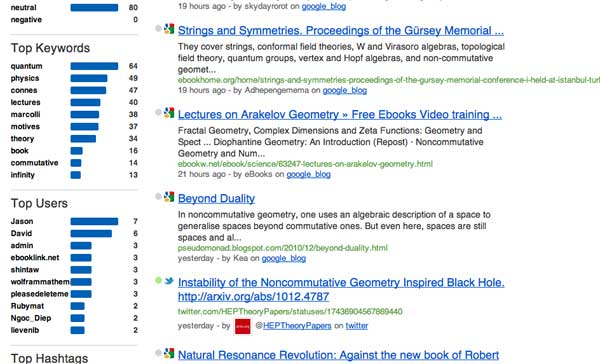SocialMention gives a rather accurate picture of the web-buzz on a specific topic. For this reason I check it irregularly to know what’s going on in noncommutative geometry, at least web-wise.

Yesterday, I noticed two new kids on the block : Jason and David. Their blogs have (so far ) 44 resp. 27 posts, this month alone. My first reaction was: respect!, until I glanced at their content…

David of E-Infinity

Noncommutative geometry has a deplorable track record when it comes to personality-cults and making extra-ordinary claims, but this site beats everything I’ve seen before. Its main mission is to spread the gospel according to E.N.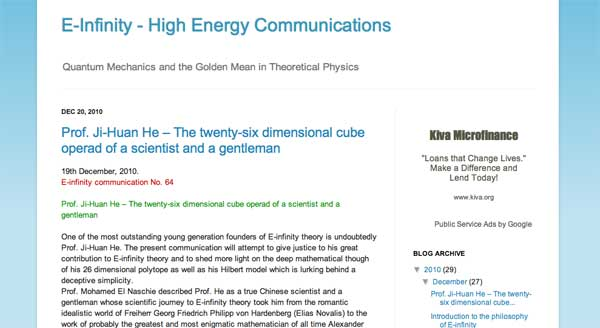A characteristic quote :

“It was no doubt the intention of those well known internet thugs and parasites to distract us from science and derail us from our road. This was the brief given to them by you know who. Never the less we will attempt to give here what can only amount to a summary of the summary of what E. N. considers to be the philosophical background to his theory.”

Jason of the E.N. watch

The blog’s mission statement is to expose the said prophet E.N. as a charlatan.The language used brings us back to the good(?!) old string-war days.

“This is amusing because E. N.’s sockpuppets go on and on about E. N. being a genius polymath with an expert grasp of science, art, history, philosophy and politics. E. N. Watch readers of course know that his knowledge in all areas comes primarily from mass-market popularizations.”

As long as the Connes support-blog and the Rosenberg support-blog remain silent and the Jasons and Davids of this world run the online ncg-show, it is probably better to drop the topic here.

No christmas- or new-years family party without heated discussions. Often on quite silly topics.

For example, which late 19th-century bookcharacter turned out to be most influential in the 20th century? Dracula, from the 1897 novel by Irish author Bram Stoker or Sir Arthur Conan Doyle’s Sherlock Holmes who made his first appearance in 1887?

Well, this year you can spice up such futile discussions by going over to Google Labs Books Ngram Viewer, specify the time period of interest to you and the relevant search terms and in no time it spits back a graph comparing the number of books mentioning these terms.

Here’s the 20th-century graph for ‘Dracula’ (blue), compared to ‘Sherlock Holmes’ (red).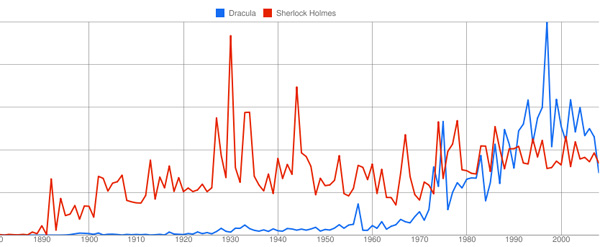The verdict being that Sherlock was the more popular of the two for the better part of the century, but in the end the vampire bit the detective. Such graphs lead to lots of new questions, such as : why was Holmes so popular in the early 30ties? and in WW2? why did Dracula become popular in the late 90ties? etc. etc.

Clearly, once you’ve used Books Ngram it’s a dangerous time-waster. Below, the graphs in the time-frame 1980-2008 for Alain Connes (blue), noncommutative geometry (red), Hopf algebras (green) and quantum groups (yellow).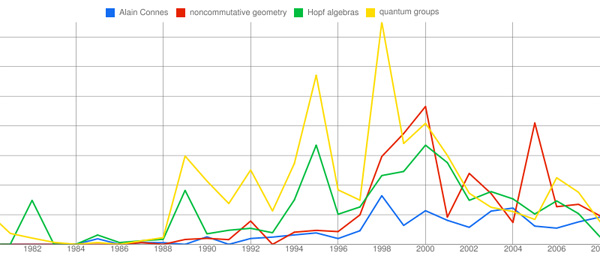It illustrates the simultaneous rise and fall of both quantum groups and Hopf algebras, whereas the noncommutative geometry-graph follows that of Alain Connes with a delay of about 2 years. I’m sure you’ll find a good use for this splendid tool…

This is a belated response to a Math-Overflow exchange between Thomas Riepe and Chandan Singh Dalawat asking for a possible connection between Connes’ noncommutative geometry approach to the Riemann hypothesis and the Langlands program.

Here’s the punchline : a large chunk of the Connes-Marcolli book Noncommutative Geometry, Quantum Fields and Motives can be read as an exploration of the noncommutative boundary to the Langlands program (at least for $GL_1$ and $GL_2$ over the rationals $\mathbb{Q}$).

Recall that Langlands for $GL_1$ over the rationals is the correspondence, given by the Artin reciprocity law, between on the one hand the abelianized absolute Galois group

$Gal(\overline{\mathbb{Q}}/\mathbb{Q})^{ab} = Gal(\mathbb{Q}(\mu_{\infty})/\mathbb{Q}) \simeq \hat{\mathbb{Z}}^*$

and on the other hand the connected components of the idele classes

$\mathbb{A}^{\ast}_{\mathbb{Q}}/\mathbb{Q}^{\ast} = \mathbb{R}^{\ast}_{+} \times \hat{\mathbb{Z}}^{\ast}$

The locally compact Abelian group of idele classes can be viewed as the nice locus of the horrible quotient space of adele classes $\mathbb{A}_{\mathbb{Q}}/\mathbb{Q}^{\ast}$. There is a well-defined map

$\mathbb{A}_{\mathbb{Q}}’/\mathbb{Q}^{\ast} \rightarrow \mathbb{R}_{+} \qquad (x_{\infty},x_2,x_3,\ldots) \mapsto | x_{\infty} | \prod | x_p |_p$

from the subset $\mathbb{A}_{\mathbb{Q}}’$ consisting of adeles of which almost all terms belong to $\mathbb{Z}_p^{\ast}$. The inverse image of this map over $\mathbb{R}_+^{\ast}$ are precisely the idele classes $\mathbb{A}^{\ast}_{\mathbb{Q}}/\mathbb{Q}^{\ast}$. In this way one can view the adele classes as a closure, or ‘compactification’, of the idele classes.

This is somewhat reminiscent of extending the nice action of the modular group on the upper-half plane to its badly behaved action on the boundary as in the Manin-Marcolli cave post.

The topological properties of the fiber over zero, and indeed of the total space of adele classes, are horrible in the sense that the discrete group $\mathbb{Q}^*$ acts ergodically on it, due to the irrationality of $log(p_1)/log(p_2)$ for primes $p_i$. All this is explained well (in the semi-local case, that is using $\mathbb{A}_Q’$ above) in the Connes-Marcolli book (section 2.7).

In much the same spirit as non-free actions of reductive groups on algebraic varieties are best handled using stacks, such ergodic actions are best handled by the tools of noncommutative geometry. That is, one tries to get at the geometry of $\mathbb{A}_{\mathbb{Q}}/\mathbb{Q}^{\ast}$ by studying an associated non-commutative algebra, the skew-ring extension of the group-ring of the adeles by the action of $\mathbb{Q}^*$ on it. This algebra is known to be Morita equivalent to the Bost-Connes algebra which is the algebra featuring in Connes’ approach to the Riemann hypothesis.

It shouldn’t thus come as a major surprise that one is able to recover the other side of the Langlands correspondence, that is the Galois group $Gal(\mathbb{Q}(\mu_{\infty})/\mathbb{Q})$, from the Bost-Connes algebra as the symmetries of certain states.

In a similar vein one can read the Connes-Marcolli $GL_2$-system (section 3.7 of their book) as an exploration of the noncommutative closure of the Langlands-space $GL_2(\mathbb{A}_{\mathbb{Q}})/GL_2(\mathbb{Q})$.

At the moment I’m running a master-seminar noncommutative geometry trying to explain this connection in detail. But, we’re still in the early phases, struggling with the topology of ideles and adeles, reciprocity laws, L-functions and the lot. Still, if someone is interested I might attempt to post some lecture notes here.

Here’s a tiny problem illustrating our limited knowledge of finite fields : “Imagine an infinite queue of Knights ${ K_1,K_2,K_3,\ldots }$, waiting to be seated at the unit-circular table. The master of ceremony (that is, you) must give Knights $K_a$ and $K_b$ a place at an odd root of unity, say $\omega_a$ and $\omega_b$, such that the seat at the odd root of unity $\omega_a \times \omega_b$ must be given to the Knight $K_{a \otimes b}$, where $a \otimes b$ is the Nim-multiplication of $a$ and $b$. Which place would you offer to Knight $K_{16}$, or Knight $K_n$, or, if you’re into ordinals, Knight $K_{\omega}$?”

What does this have to do with finite fields? Well, consider the simplest of all finite field $\mathbb{F}_2 = { 0,1 }$ and consider its algebraic closure $\overline{\mathbb{F}_2}$. Last year, we’ve run a series starting here, identifying the field $\overline{\mathbb{F}_2}$, following John H. Conway in ONAG, with the set of all ordinals smaller than $\omega^{\omega^{\omega}}$, given the Nim addition and multiplication. I know that ordinal numbers may be intimidating at first, so let’s just restrict to ordinary natural numbers for now. The Nim-addition of two numbers $n \oplus m$ can be calculated by writing the numbers n and m in binary form and add them without carrying. For example, $9 \oplus 1 = 1001+1 = 1000 = 8$. Nim-multiplication is slightly more complicated and is best expressed using the so-called Fermat-powers $F_n = 2^{2^n}$. We then demand that $F_n \otimes m = F_n \times m$ whenever $m < F_n$ and $F_n \otimes F_n = \frac{3}{2}F_n$. Distributivity wrt. $\oplus$ can then be used to calculate arbitrary Nim-products. For example, $8 \otimes 3 = (4 \otimes 2) \otimes (2 \oplus 1) = (4 \otimes 3) \oplus (4 \otimes 2) = 12 \oplus 8 = 4$. Conway’s remarkable result asserts that the ordinal numbers, equipped with Nim addition and multiplication, form an algebraically closed field of characteristic two. The closure $\overline{\mathbb{F}_2}$ is identified with the subfield of all ordinals smaller than $\omega^{\omega^{\omega}}$. For those of you who don’t feel like going transfinite, the subfield $~(\mathbb{N},\oplus,\otimes)$ is identified with the quadratic closure of $\mathbb{F}_2$.

The connection between $\overline{\mathbb{F}_2}$ and the odd roots of unity has been advocated by Alain Connes in his talk before a general public at the IHES : “L’ange de la géométrie, le diable de l’algèbre et le corps à un élément” (the angel of geometry, the devil of algebra and the field with one element). He describes its content briefly in this YouTube-video

At first it was unclear to me which ‘coupling-problem’ Alain meant, but this has been clarified in his paper together with Caterina Consani Characteristic one, entropy and the absolute point. The non-zero elements of $\overline{\mathbb{F}_2}$ can be identified with the set of all odd roots of unity. For, if x is such a unit, it belongs to a finite subfield of the form $\mathbb{F}_{2^n}$ for some n, and, as the group of units of any finite field is cyclic, x is an element of order $2^n-1$. Hence, $\mathbb{F}_{2^n}- { 0 }$ can be identified with the set of $2^n-1$-roots of unity, with $e^{2 \pi i/n}$ corresponding to a generator of the unit-group. So, all elements of $\overline{\mathbb{F}_2}$ correspond to an odd root of unity. The observation that we get indeed all odd roots of unity may take you a couple of seconds (( If m is odd, then (2,m)=1 and so 2 is a unit in the finite cyclic group $~(\mathbb{Z}/m\mathbb{Z})^*$ whence $2^n = 1 (mod~m)$, so the m-roots of unity lie within those of order $2^n-1$ )).

Assuming we succeed in fixing a one-to-one correspondence between the non-zero elements of $\overline{\mathbb{F}_2}$ and the odd roots of unity $\mu_{odd}$ respecting multiplication, how can we recover the addition on $\overline{\mathbb{F}_2}$? Well, here’s Alain’s coupling function, he ties up an element x of the algebraic closure to the element s(x)=x+1 (and as we are in characteristic two, this is an involution, so also the element tied up to x+1 is s(x+1)=(x+1)+1=x. The clue being that multiplication together with the coupling map s allows us to compute any sum of two elements as $x+y=x \times s(\frac{y}{x}) = x \times (\frac{y}{x}+1)$.
For example, all information about the finite field $\mathbb{F}_{2^4}$ is encoded in this identification with the 15-th roots of unity, together with the pairing s depicted as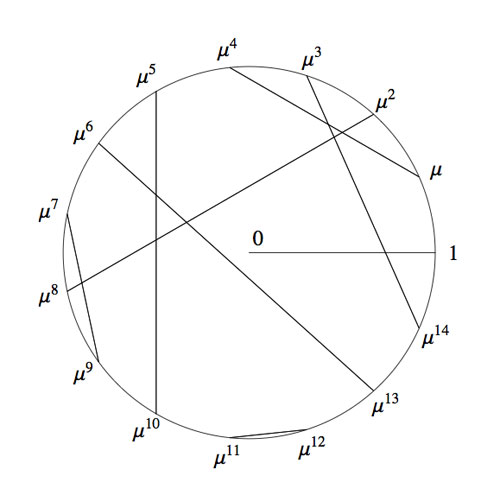Okay, we now have two identifications of the algebraic closure $\overline{\mathbb{F}_2}$ : the smaller ordinals equipped with Nim addition and Nim multiplication and the odd roots of unity with complex-multiplication and the Connes-coupling s. The question we started from asks for a general recipe to identify these two approaches.

To those of you who are convinced that finite fields (LOL, even characteristic two!) are objects far too trivial to bother thinking about : as far as I know, NOBODY knows how to do this explicitly, even restricting the ordinals to merely the natural numbers!

Please feel challenged! To get you started, I’ll show you how to place the first 15 Knights and give you a procedure (though far from explicit) to continue. Here’s the Nim-picture compatible with that above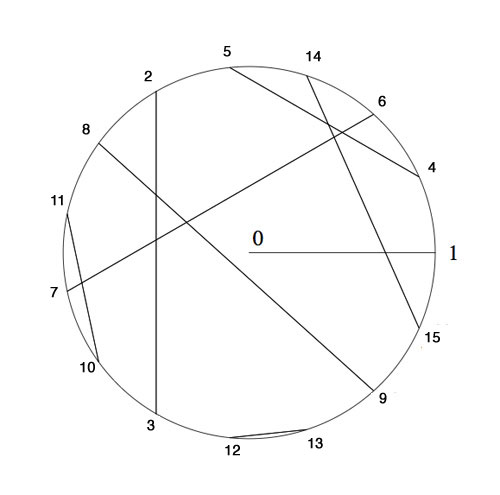To verify this, and to illustrate the general strategy, I’d better hand you the Nim-tables of the first 16 numbers. Here they are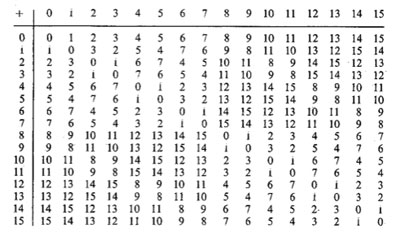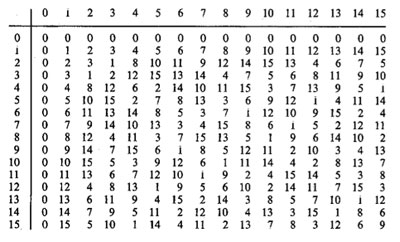It is known that the finite subfields of $~(\mathbb{N},\oplus,\otimes)$ are precisely the sets of numbers smaller than the Fermat-powers $F_n$. So, the first one is all numbers smaller than $F_1=4$ (check!). The smallest generator of the multiplicative group (of order 3) is 2, so we take this to correspond to the unit-root $e^{2 \pi i/3}$. The next subfield are all numbers smaller than $F_2 = 16$ and its multiplicative group has order 15. Now, choose the smallest integer k which generates this group, compatible with the condition that $k^{\otimes 5}=2$. Verify that this number is 4 and that this forces the identification and coupling given above.

The next finite subfield would consist of all natural numbers smaller than $F_3=256$. Hence, in this field we are looking for the smallest number k generating the multiplicative group of order 255 satisfying the extra condition that $k^{\otimes 17}=4$ which would fix an identification at that level. Then, the next level would be all numbers smaller than $F_4=65536$ and again we would like to find the smallest number generating the multiplicative group and such that the appropriate power is equal to the aforementioned k, etc. etc.

Can you give explicit (even inductive) formulae to achieve this? I guess even the problem of placing Knight 16 will give you a couple of hours to think about… (to be continued).

Today, Alain Connes and Caterina Consani arXived their new paper Schemes over $\mathbb{F}_1$ and zeta functions. It is a follow-up to their paper On the notion of geometry over $\mathbb{F}_1$, which I’ve tried to explain in a series of posts starting here.

As Javier noted already last week when they updated their first paper, the main point of the first 25 pages of the new paper is to repace abelian groups by abelian monoids in the definition, making it more in tune with other approaches, most notably that of Anton Deitmar. The novelty, if you want, is that they package the two functors $\mathbf{rings} \rightarrow \mathbf{sets}$ and $\mathbf{ab-monoid} \rightarrow \mathbf{sets}$ into one functor $\mathbf{ring-monoid} \rightarrow \mathbf{sets}$ by using the ‘glued category’ $\mathbf{ring-monoid}$ (an idea they attribute to Pierre Cartier).

In general, if you have two categories $\mathbf{cat}$ and $\mathbf{cat’}$ and a pair of adjoint functors between them, then one can form the glued-category $\mathbf{cat-cat’}$ by taking as its collection of objects the disjoint union of the objects of the two categories and by defining the hom-sets between two objects the hom-sets in either category (if both objects belong to the same category) or use the adjoint functors to define the new hom-set when they do not (the very definition of adjoint functors makes that this doesn’t depend on the choice).

Here, one uses the functor $\mathbf{ab-monoid} \rightarrow \mathbf{rings}$ assigning to a monoid $M$ its integral monoid-algebra $\mathbb{Z}[M]$, having as its adjoint the functor $\mathbf{rings} \rightarrow \mathbf{ab-monoid}$ forgetting the additive structure of the commutative ring.

In the second part of the paper, they first prove some nice results on zeta-functions of Noetherian $\mathbb{F}_1$-schemes and extend them, somewhat surprisingly, to settings which do not (yet) fit into the $\mathbb{F}_1$-framework, namely elliptic curves and the hypothetical $\mathbb{F}_1$-curve $\overline{\mathbf{spec}(\mathbb{Z})}$.

In ONAG, John Conway proves that the symmetric version of his recursive definition of addition and multiplcation on the surreal numbers make the class On of all Cantor’s ordinal numbers into an algebraically closed Field of characteristic two : On2 (pronounced ‘Onto’), and, in particular, he identifies a subfield
with the algebraic closure of the field of two elements. What makes all of this somewhat confusing is that Cantor had already defined a (badly behaving) addition, multiplication and exponentiation on ordinal numbers.

Over the last week I’ve been playing a bit with sage to prove a few exotic identities involving ordinal numbers. Here’s one of them ($\omega$ is the first infinite ordinal number, that is, $\omega={ 0,1,2,\ldots }$),

$~(\omega^{\omega^{13}})^{47} = \omega^{\omega^7} + 1$

answering a question in Hendrik Lenstra’s paper Nim multiplication.

However, it will take us a couple of posts before we get there. Let’s begin by trying to explain what brought this on. On september 24th 2008 there was a meeting, intended for a general public, called a la rencontre des dechiffeurs, celebrating the 50th birthday of the IHES.

One of the speakers was Alain Connes and the official title of his talk was “L’ange de la géométrie, le diable de l’algèbre et le corps à un élément” (the angel of geometry, the devil of algebra and the field with one element). Instead, he talked about a seemingly trivial problem : what is the algebraic closure of $\mathbb{F}_2$, the field with two elements? My only information about the actual content of the talk comes from the following YouTube-blurb

Alain argues that we do not have a satisfactory description of $\overline{\mathbb{F}}_2$, the algebraic closure of $\mathbb{F}_2$. Naturally, it is the union (or rather, limit) of all finite fields $\mathbb{F}_{2^n}$, but, there are too many non-canonical choices to make here.

Recall that $\mathbb{F}_{2^k}$ is a subfield of $\mathbb{F}_{2^l}$ if and only if $k$ is a divisor of $l$ and so we would have to take the direct limit over the integers with respect to the divisibility relation… Of course, we can replace this by an increasing sequence of a selection of cofinal fields such as

$\mathbb{F}_{2^{1!}} \subset \mathbb{F}_{2^{2!}} \subset \mathbb{F}_{2^{3!}} \subset \ldots$

But then, there are several such suitable sequences! Another ambiguity comes from the description of $\mathbb{F}_{2^n}$. Clearly it is of the form $\mathbb{F}_2[x]/(f(x))$ where $f(x)$ is a monic irreducible polynomial of degree $n$, but again, there are several such polynomials. An attempt to make a canonical choice of polynomial is to take the ‘first’ suitable one with respect to some natural ordering on the polynomials. This leads to the so called Conway polynomials.

Conway polynomials for the prime $2$ have only been determined up to degree 400-something, so in the increasing sequence above we would already be stuck at the sixth term $\mathbb{F}_{2^{6!}}$…

So, what Alain Connes sets as a problem is to find another, more canonical, description of $\overline{\mathbb{F}}_2$. The problem is not without real-life interest as most finite fields appearing in cryptography or coding theory are subfields of $\overline{\mathbb{F}}_2$.

(My guess is that Alain originally wanted to talk about the action of the Galois group on the roots of unity, which would be the corresponding problem over the field with one element and would explain the title of the talk, but decided against it. If anyone knows what ‘coupling-problem’ he is referring to, please drop a comment.)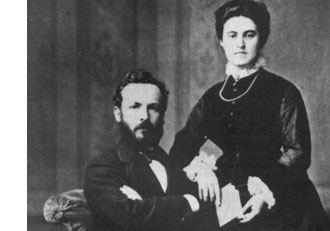Surely, Connes is aware of the fact that there exists a nice canonical recursive construction of $\overline{\mathbb{F}}_2$ due to John Conway, using Georg Cantor’s ordinal numbers.

In fact, in chapter 6 of his book On Numbers And Games, John Conway proves that the symmetric version of his recursive definition of addition and multiplcation on the surreal numbers make the class $\mathbf{On}$ of all Cantor’s ordinal numbers into an algebraically closed Field of characteristic two : $\mathbf{On}_2$ (pronounced ‘Onto’), and, in particular, he identifies a subfield

$\overline{\mathbb{F}}_2 \simeq [ \omega^{\omega^{\omega}} ]$

with the algebraic closure of $\mathbb{F}_2$. What makes all of this somewhat confusing is that Cantor had already defined a (badly behaving) addition, multiplication and exponentiation on ordinal numbers. To distinguish between the Cantor/Conway arithmetics, Conway (and later Lenstra) adopt the convention that any expression between square brackets refers to Cantor-arithmetic and un-squared ones to Conway’s. So, in the description of the algebraic closure just given $[ \omega^{\omega^{\omega}} ]$ is the ordinal defined by Cantor-exponentiation, whereas the exotic identity we started out with refers to Conway’s arithmetic on ordinal numbers.

Let’s recall briefly Cantor’s ordinal arithmetic. An ordinal number $\alpha$ is the order-type of a totally ordered set, that is, if there is an order preserving bijection between two totally ordered sets then they have the same ordinal number (or you might view $\alpha$ itself as a totally ordered set, namely the set of all strictly smaller ordinal numbers, so e.g. $0= \emptyset,1= { 0 },2={ 0,1 },\ldots$).

For two ordinals $\alpha$ and $\beta$, the addition $[\alpha + \beta ]$ is the order-type of the totally ordered set $\alpha \sqcup \beta$ (the disjoint union) ordered compatible with the total orders in $\alpha$ and $\beta$ and such that every element of $\beta$ is strictly greater than any element from $\alpha$. Observe that this definition depends on the order of the two factors. For example,$[1 + \omega] = \omega$ as there is an order preserving bijection ${ \tilde{0},0,1,2,\ldots } \rightarrow { 0,1,2,3,\ldots }$ by $\tilde{0} \mapsto 0,n \mapsto n+1$. However, $\omega \not= [\omega + 1]$ as there can be no order preserving bijection ${ 0,1,2,\ldots } \rightarrow { 0,1,2,\ldots,0_{max} }$ as the first set has no maximal element whereas the second one does. So, Cantor’s addition has the bad property that it may be that $[\alpha + \beta] \not= [\beta + \alpha]$.

The Cantor-multiplication $\alpha . \beta$ is the order-type of the product-set $\alpha \times \beta$ ordered via the last differing coordinate. Again, this product has the bad property that it may happen that $[\alpha . \beta] \not= [\beta . \alpha]$ (for example $[2 . \omega ] \not=[ \omega . 2 ]$). Finally, the exponential $\beta^{\alpha}$ is the order type of the set of all maps $f~:~\alpha \rightarrow \beta$ such that $f(a) \not=0$ for only finitely many $a \in \alpha$, and ordered via the last differing function-value.

Cantor’s arithmetic allows normal-forms for ordinal numbers. More precisely, with respect to any ordinal number $\gamma \geq 2$, every ordinal number $\alpha \geq 1$ has a unique expression as

$\alpha = [ \gamma^{\alpha_0}.\eta_0 + \gamma^{\alpha_1}.\eta_1 + \ldots + \gamma^{\alpha_m}.\eta_m]$

for some natural number $m$ and such that $\alpha \geq \alpha_0 > \alpha_1 > \ldots > \alpha_m \geq 0$ and all $1 \leq \eta_i < \gamma$. In particular, taking the special cases $\gamma = 2$ and $\gamma = \omega$, we have the following two canonical forms for any ordinal number $\alpha$

$[ 2^{\alpha_0} + 2^{\alpha_1} + \ldots + 2^{\alpha_m}] = \alpha = [ \omega^{\beta_0}.n_0 + \omega^{\beta_1}.n_1 + \ldots + \omega^{\beta_k}.n_k]$

with $m,k,n_i$ natural numbers and $\alpha \geq \alpha_0 > \alpha_1 > \ldots > \alpha_m \geq 0$ and $\alpha \geq \beta_0 > \beta_1 > \ldots > \beta_k \geq 0$. Both canonical forms will be important when we consider the (better behaved) Conway-arithmetic on $\mathbf{On}_2$, next time.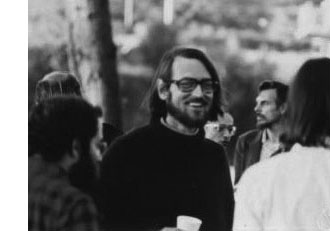David Mumford did receive earlier this year the 2007 AMS Leroy P. Steele Prize for Mathematical Exposition. The jury honors Mumford for “his beautiful expository accounts of a host of aspects of algebraic geometry”. Not surprisingly, the first work they mention are his mimeographed notes of the first 3 chapters of a course in algebraic geometry, usually called “Mumford’s red book” because the notes were wrapped in a red cover. In 1988, the notes were reprinted by Springer-Verlag. Unfortnately, the only red they preserved was in the title.

The AMS describes the importance of the red book as follows. “This is one of the few books that attempt to convey in pictures some of the highly abstract notions that arise in the field of algebraic geometry. In his response upon receiving the prize, Mumford recalled that some of his drawings from The Red Book were included in a collection called Five Centuries of French Mathematics. This seemed fitting, he noted: “After all, it was the French who started impressionist painting and isn’t this just an impressionist scheme for rendering geometry?””

These days it is perfectly possible to get a good grasp on difficult concepts from algebraic geometry by reading blogs, watching YouTube or plugging in equations to sophisticated math-programs. In the early seventies though, if you wanted to know what Grothendieck’s scheme-revolution was all about you had no choice but to wade through the EGA’s and SGA’s and they were notorious for being extremely user-unfriendly regarding illustrations…

So the few depictions of schemes available, drawn by people sufficiently fluent in Grothendieck’s new geometric language had no less than treasure-map-cult-status and were studied in minute detail. Mumford’s red book was a gold mine for such treasure maps. Here’s my favorite one, scanned from the original mimeographed notes (it looks somewhat tidier in the Springer-version)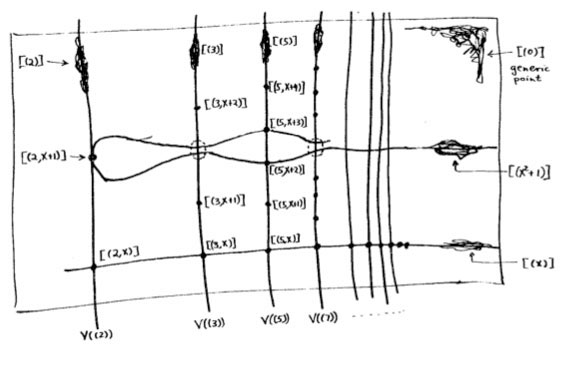It is the first depiction of $\mathbf{spec}(\mathbb{Z}[x])$, the affine scheme of the ring $\mathbb{Z}[x]$ of all integral polynomials. Mumford calls it the”arithmetic surface” as the picture resembles the one he made before of the affine scheme $\mathbf{spec}(\mathbb{C}[x,y])$ corresponding to the two-dimensional complex affine space $\mathbb{A}^2_{\mathbb{C}}$. Mumford adds that the arithmetic surface is ‘the first example which has a real mixing of arithmetic and geometric properties’.

Let’s have a closer look at the treasure map. It introduces some new signs which must have looked exotic at the time, but have since become standard tools to depict algebraic schemes.

For starters, recall that the underlying topological space of $\mathbf{spec}(\mathbb{Z}[x])$ is the set of all prime ideals of the integral polynomial ring $\mathbb{Z}[x]$, so the map tries to list them all as well as their inclusions/intersections.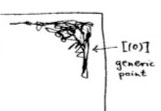The doodle in the right upper corner depicts the ‘generic point’ of the scheme. That is, the geometric object corresponding to the prime ideal $~(0)$ (note that $\mathbb{Z}[x]$ is an integral domain). Because the zero ideal is contained in any other prime ideal, the algebraic/geometric mantra (“inclusions reverse when shifting between algebra and geometry”) asserts that the gemetric object corresponding to $~(0)$ should contain all other geometric objects of the arithmetic plane, so it is just the whole plane! Clearly, it is rather senseless to depict this fact by coloring the whole plane black as then we wouldn’t be able to see the finer objects. Mumford’s solution to this is to draw a hairy ball, which in this case, is sufficiently thick to include fragments going in every possible direction. In general, one should read these doodles as saying that the geometric object represented by this doodle contains all other objects seen elsewhere in the picture if the hairy-ball-doodle includes stuff pointing in the direction of the smaller object. So, in the case of the object corresponding to $~(0)$, the doodle has pointers going everywhere, saying that the geometric object contains all other objects depicted.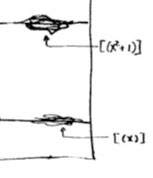Let’s move over to the doodles in the lower right-hand corner. They represent the geometric object corresponding to principal prime ideals of the form $~(p(x))$, where $p(x)$ in an irreducible polynomial over the integers, that is, a polynomial which we cannot write as the product of two smaller integral polynomials. The objects corresponding to such prime ideals should be thought of as ‘horizontal’ curves in the plane.

The doodles depicted correspond to the prime ideal $~(x)$, containing all polynomials divisible by $x$ so when we divide it out we get, as expected, a domain $\mathbb{Z}[x]/(x) \simeq \mathbb{Z}$, and the one corresponding to the ideal $~(x^2+1)$, containing all polynomials divisible by $x^2+1$, which can be proved to be a prime ideals of $\mathbb{Z}[x]$ by observing that after factoring out we get $\mathbb{Z}[x]/(x^2+1) \simeq \mathbb{Z}[i]$, the domain of all Gaussian integers $\mathbb{Z}[i]$. The corresponding doodles (the ‘generic points’ of the curvy-objects) have a predominant horizontal component as they have the express the fact that they depict horizontal curves in the plane. It is no coincidence that the doodle of $~(x^2+1)$ is somewhat bulkier than the one of $~(x)$ as the later one must only depict the fact that all points lying on the straight line to its left belong to it, whereas the former one must claim inclusion of all points lying on the ‘quadric’ it determines.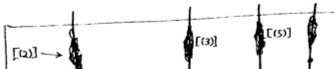Apart from these ‘horizontal’ curves, there are also ‘vertical’ lines corresponding to the principal prime ideals $~(p)$, containing the polynomials, all of which coefficients are divisible by the prime number $p$. These are indeed prime ideals of $\mathbb{Z}[x]$, because their quotients are
$\mathbb{Z}[x]/(p) \simeq (\mathbb{Z}/p\mathbb{Z})[x]$ are domains, being the ring of polynomials over the finite field $\mathbb{Z}/p\mathbb{Z} = \mathbb{F}_p$. The doodles corresponding to these prime ideals have a predominant vertical component (depicting the ‘vertical’ lines) and have a uniform thickness for all prime numbers $p$ as each of them only has to claim ownership of the points lying on the vertical line under them.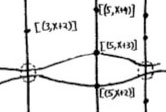Right! So far we managed to depict the zero prime ideal (the whole plane) and the principal prime ideals of $\mathbb{Z}[x]$ (the horizontal curves and the vertical lines). Remains to depict the maximal ideals. These are all known to be of the form
$\mathfrak{m} = (p,f(x))$
where $p$ is a prime number and $f(x)$ is an irreducible integral polynomial, which remains irreducible when reduced modulo $p$ (that is, if we reduce all coefficients of the integral polynomial $f(x)$ modulo $p$ we obtain an irreducible polynomial in $~\mathbb{F}_p[x]$). By the algebra/geometry mantra mentioned before, the geometric object corresponding to such a maximal ideal can be seen as the ‘intersection’ of an horizontal curve (the object corresponding to the principal prime ideal $~(f(x))$) and a vertical line (corresponding to the prime ideal $~(p)$). Because maximal ideals do not contain any other prime ideals, there is no reason to have a doodle associated to $\mathfrak{m}$ and we can just depict it by a “point” in the plane, more precisely the intersection-point of the horizontal curve with the vertical line determined by $\mathfrak{m}=(p,f(x))$. Still, Mumford’s treasure map doesn’t treat all “points” equally. For example, the point corresponding to the maximal ideal $\mathfrak{m}_1 = (3,x+2)$ is depicted by a solid dot $\mathbf{.}$, whereas the point corresponding to the maximal ideal $\mathfrak{m}_2 = (3,x^2+1)$ is represented by a fatter point $\circ$. The distinction between the two ‘points’ becomes evident when we look at the corresponding quotients (which we know have to be fields). We have

$\mathbb{Z}[x]/\mathfrak{m}_1 = \mathbb{Z}[x]/(3,x+2)=(\mathbb{Z}/3\mathbb{Z})[x]/(x+2) = \mathbb{Z}/3\mathbb{Z} = \mathbb{F}_3$ whereas $\mathbb{Z}[x]/\mathfrak{m}_2 = \mathbb{Z}[x]/(3,x^2+1) = \mathbb{Z}/3\mathbb{Z}[x]/(x^2+1) = \mathbb{F}_3[x]/(x^2+1) = \mathbb{F}_{3^2}$

because the polynomial $x^2+1$ remains irreducible over $\mathbb{F}_3$, the quotient $\mathbb{F}_3[x]/(x^2+1)$ is no longer the prime-field $\mathbb{F}_3$ but a quadratic field extension of it, that is, the finite field consisting of 9 elements $\mathbb{F}_{3^2}$. That is, we represent the ‘points’ lying on the vertical line corresponding to the principal prime ideal $~(p)$ by a solid dot . when their quotient (aka residue field is the prime field $~\mathbb{F}_p$, by a bigger point $\circ$ when its residue field is the finite field $~\mathbb{F}_{p^2}$, by an even fatter point $\bigcirc$ when its residue field is $~\mathbb{F}_{p^3}$ and so on, and on. The larger the residue field, the ‘fatter’ the corresponding point.

In fact, the ‘fat-point’ signs in Mumford’s treasure map are an attempt to depict the fact that an affine scheme contains a lot more information than just the set of all prime ideals. In fact, an affine scheme determines (and is determined by) a “functor of points”. That is, to every field (or even every commutative ring) the affine scheme assigns the set of its ‘points’ defined over that field (or ring). For example, the $~\mathbb{F}_p$-points of $\mathbf{spec}(\mathbb{Z}[x])$ are the solid . points on the vertical line $~(p)$, the $~\mathbb{F}_{p^2}$-points of $\mathbf{spec}(\mathbb{Z}[x])$ are the solid . points and the slightly bigger $\circ$ points on that vertical line, and so on.

This concludes our first attempt to decypher Mumford’s drawing, but if we delve a bit deeper, we are bound to find even more treasures… (to be continued).

Last time we tried to generalize the Connes-Consani approach to commutative algebraic geometry over the field with one element $\mathbb{F}_1$ to the noncommutative world by considering covariant functors

$N~:~\mathbf{groups} \rightarrow \mathbf{sets}$

which over $\mathbb{C}$ resp. $\mathbb{Z}$ become visible by a complex (resp. integral) algebra having suitable universal properties.

However, we didn’t specify what we meant by a complex noncommutative variety (resp. an integral noncommutative scheme). In particular, we claimed that the $\mathbb{F}_1$-‘points’ associated to the functor

$D~:~\mathbf{groups} \rightarrow \mathbf{sets} \qquad G \mapsto G_2 \times G_3$ (here $G_n$ denotes all elements of order $n$ of $G$)

were precisely the modular dessins d’enfants of Grothendieck, but didn’t give details. We’ll try to do this now.

For algebras over a field we follow the definition, due to Kontsevich and Soibelman, of so called “noncommutative thin schemes”. Actually, the thinness-condition is implicit in both Soule’s-approach as that of Connes and Consani : we do not consider R-points in general, but only those of rings R which are finite and flat over our basering (or field).

So, what is a noncommutative thin scheme anyway? Well, its a covariant functor (commuting with finite projective limits)

$\mathbb{X}~:~\mathbf{Alg}^{fd}_k \rightarrow \mathbf{sets}$

from finite-dimensional (possibly noncommutative) $k$-algebras to sets. Now, the usual dual-space operator gives an anti-equivalence of categories

$\mathbf{Alg}^{fd}_k \leftrightarrow \mathbf{Coalg}^{fd}_k \qquad A=C^* \leftrightarrow C=A^*$

so a thin scheme can also be viewed as a contra-variant functor (commuting with finite direct limits)

$\mathbb{X}~:~\mathbf{Coalg}^{fd}_k \rightarrow \mathbf{Sets}$

In particular, we are interested to associated to any {tex]k $-algebra$A $its representation functor :$\mathbf{rep}(A)~:~\mathbf{Coalg}^{fd}_k \rightarrow \mathbf{Sets} \qquad C \mapsto Alg_k(A,C^*) $This may look strange at first sight, but$C^* $is a finite dimensional algebra and any$n $-dimensional representation of$A $is an algebra map$A \rightarrow M_n(k) $and we take$C $to be the dual coalgebra of this image. Kontsevich and Soibelman proved that every noncommutative thin scheme$\mathbb{X} $is representable by a$k $-coalgebra. That is, there exists a unique coalgebra$C_{\mathbb{X}} $(which they call the coalgebra of ‘distributions’ of$\mathbb{X} $) such that for every finite dimensional$k $-algebra$B $we have$\mathbb{X}(B) = Coalg_k(B^*,C_{\mathbb{X}}) $In the case of interest to us, that is for the functor$\mathbf{rep}(A) $the coalgebra of distributions is Kostant’s dual coalgebra$A^o $. This is the not the full linear dual of$A $but contains only those linear functionals on$A $which factor through a finite dimensional quotient. So? You’ve exchanged an algebra$A $for some coalgebra$A^o $, but where’s the geometry in all this? Well, let’s look at the commutative case. Suppose$A= \mathbb{C}[X] $is the coordinate ring of a smooth affine variety$X $, then its dual coalgebra looks like$\mathbb{C}[X]^o = \oplus_{x \in X} U(T_x(X)) $the direct sum of all universal (co)algebras of tangent spaces at points$x \in X $. But how do we get the variety out of this? Well, any coalgebra has a coradical (being the sun of all simple subcoalgebras) and in the case just mentioned we have$corad(\mathbb{C}[X]^o) = \oplus_{x \in X} \mathbb{C} e_x $so every point corresponds to a unique simple component of the coradical. In the general case, the coradical of the dual coalgebra$A^o $is the direct sum of all simple finite dimensional representations of$A $. That is, the direct summands of the coalgebra give us a noncommutative variety whose points are the simple representations, and the remainder of the coalgebra of distributions accounts for infinitesimal information on these points (as do the tangent spaces in the commutative case). In fact, it was a surprise to me that one can describe the dual coalgebra quite explicitly, and that$A_{\infty} $-structures make their appearance quite naturally. See this paper if you’re in for the details on this. That settles the problem of what we mean by the noncommutative variety associated to a complex algebra. But what about the integral case? In the above, we used extensively the theory of Kostant-duality which works only for algebras over fields… Well, not quite. In the case of$\mathbb{Z} $(or more general, of Dedekind domains) one can repeat Kostant’s proof word for word provided one takes as the definition of the dual$\mathbb{Z} $-coalgebra of an algebra (which is$\mathbb{Z} $-torsion free)$A^o = { f~:~A \rightarrow \mathbb{Z}~:~A/Ker(f)~\text{is finitely generated and torsion free}~} $(over general rings there may be also variants of this duality, as in Street’s book an Quantum groups). Probably lots of people have come up with this, but the only explicit reference I have is to the first paper I’ve ever written. So, also for algebras over$\mathbb{Z} $we can define a suitable noncommutative integral scheme (the coradical approach accounts only for the maximal ideals rather than all primes, but somehow this is implicit in all approaches as we consider only thin schemes). Fine! So, we can make sense of the noncommutative geometrical objects corresponding to the group-algebras$\mathbb{C} \Gamma $and$\mathbb{Z} \Gamma $where$\Gamma = PSL_2(\mathbb{Z}) $is the modular group (the algebras corresponding to the$G \mapsto G_2 \times G_3 $-functor). But, what might be the points of the noncommutative scheme corresponding to$\mathbb{F}_1 \Gamma $??? Well, let’s continue the path cut out before. “Points” should correspond to finite dimensional “simple representations”. Hence, what are the finite dimensional simple$\mathbb{F}_1 $-representations of$\Gamma $? (Or, for that matter, of any group$G $) Here we come back to Javier’s post on this : a finite dimensional$\mathbb{F}_1 $-vectorspace is a finite set. A$\Gamma $-representation on this set (of n-elements) is a group-morphism$\Gamma \rightarrow GL_n(\mathbb{F}_1) = S_n $hence it gives a permutation representation of$\Gamma $on this set. But then, if finite dimensional$\mathbb{F}_1 $-representations of$\Gamma $are the finite permutation representations, then the simple ones are the transitive permutation representations. That is, the points of the noncommutative scheme corresponding to$\mathbb{F}_1 \Gamma $are the conjugacy classes of subgroups$H \subset \Gamma $such that$\Gamma/H \$ is finite. But these are exactly the modular dessins d’enfants introduced by Grothendieck as I explained a while back elsewhere (see for example this post and others in the same series).